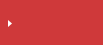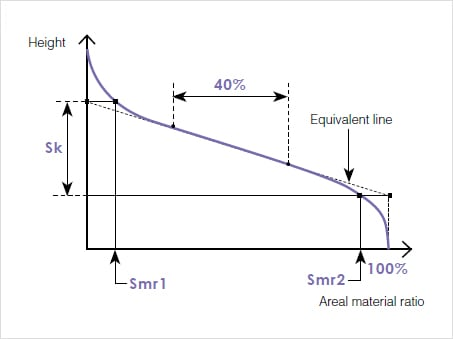>  What is area roughness?  >  Area Roughness Parameters

# Sk(Core height) | Area Roughness Parameters

## surface texture parameters in ISO 25178

• Height
• Spatial
• Hybrid
• Functional
• Functional volume
• FeatureSearch from the parameters list

## Sk(Core height)

Sk(Core height) is calculated as the difference of heights at the areal material ratio values 0% and 100% on the equivalent line; specifically, it is a value obtained by subtracting the minimum height from the maximum height of the core surface.Sk represents the height of the core surface, Smr1 represents the areal material ratio that divides the reduced peaks from the core surface, and Smr2 represents the areal material ratio that divides the reduced valleys from the core surface.

The reduced peaks are the areas that are removed by initial abrasion. Spk represents the average height of the reduced peaks.

The reduced valleys are the areas that hold liquid applied on the surface in order to improve lubricity. Svk represents the average depth of the reduced valleys.## Search from the parameters list

Height Sa (arithmetical mean height) Sz (Maximum height) Sq (Root mean square height) Ssk (Skewness) Sku (Kurtosis) Sp (Maximum peak height) Sv (Maximum pit height) Sal (Auto-correlation length) / Str (Texture aspect ratio) Std* (Texture direction) Sdq (Root mean square gradient) Sdr (Developed interfacial area ratio) Smr(c) Areal material (bearing area) ratio Smc(mr) Inverse areal material ratio Sk(Core height) Spk (Reduced peak height) Svk (reduced dale height (reduced valley depth)) Smr (Peak material portion) Smr2 (Valley material portion) Sxp (Peak extreme height) Vvv (Dale void volume) Vvc (Core void volume) Vmp (Peak material volume) Vmc (Core material volume) Spd (Density of peaks) Spc (Arithmetic mean peak curvature) S10z / S5p / S5v / Sda(c) / Sha(c) / Sdv(c) / Shv(c)